Updated 26/09/2023
In force

Version from: 28/06/2023
Amendments
There is currently no level 2 legal act based on or specifying Article 280b.
Search within this legal act

### Article 280b - Foreign exchange risk category add-on

Article 280b

1.

For the purposes of Article 278, institutions shall calculate the foreign exchange risk category add-on for a given netting set as follows: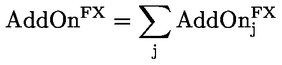where:

 j = the index that denotes the foreign exchange risk hedging sets established in accordance with point (b) of Article 277a(1) and with Article 277a(2) for the netting set; and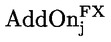= the foreign exchange risk category add-on for hedging set j calculated in accordance with paragraph 2.
2.

Institutions shall calculate the foreign exchange risk category add-on for hedging set j as follows: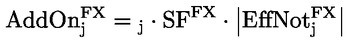where:

 єj = the hedging set supervisory factor coefficient of hedging set j determined in accordance with Article 280;
 SFFX = the supervisory factor for the foreign exchange risk category with a value equal to 4 %;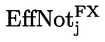= the effective notional amount of hedging set j calculated as follows: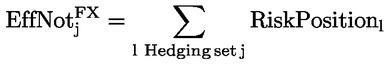where:

 l = the index that denotes the risk position.